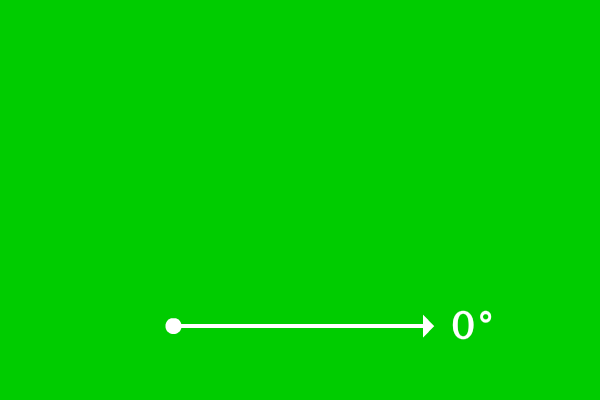# Acute angle

## Definition

An angle of less than $90^\circ$ is called an acute angleThe meaning of acute is, an angle of less than $90^\circ$. Therefore, if any angle is less than $90^\circ$, the angle is called as an acute angle geometrically.

Zero angle is a best example of an acute angle because the range of acute angle starts from zero degrees and ends just before $90^\circ$.

Mathematically, the range of an acute angle can be expressed as $[0^\circ, 90^\circ)$.

### Case study

Acute angles are geometrically formed in two different possible cases.

1

#### Acute angle by a LineAngle of any line is measured from the zero degrees because it is parallel to the surface of the earth.

$\overrightarrow{AB}$ is a ray and it is initially at $0^\circ$ angle. Later, the ray $\overrightarrow{AB}$ is rotated an angle of $56^\circ$ in anticlockwise direction to become $\overrightarrow{AC}$ at its final position.

The ray $\overrightarrow{AB}$ is made an angle of $56^\circ$ to reach its final position from its initial position.

$\angle CAB = 56^\circ$

The angle $CAB$ is less than $90^\circ$. Therefore, the $\angle CAB$ is called as an acute angle.

2

#### Acute angle between two lines$\overrightarrow{DE}$ and $\overrightarrow{DF}$ are two rays and they both started from point $D$.

The two rays have a common vertex and an angle is formed between them. The angle between them is measured by considering one ray as baseline to measure the angle of another ray and vice-versa.

The angle between them is measured as $38^\circ$.

$\angle EDF = 38^\circ$

The angle $EDF$ is less than $90^\circ$. Therefore, it is called as an acute angle geometrically.

Latest Math Topics
Jun 26, 2023
Jun 23, 2023

Latest Math Problems
Jul 01, 2023
Jun 25, 2023
###### Math Questions

The math problems with solutions to learn how to solve a problem.

Learn solutions

Practice now

###### Math Videos

The math videos tutorials with visual graphics to learn every concept.

Watch now

###### Subscribe us

Get the latest math updates from the Math Doubts by subscribing us.